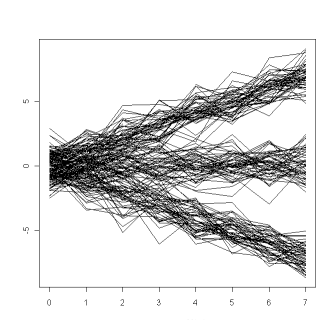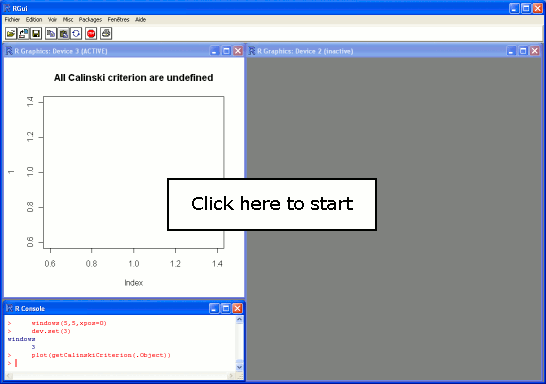﻿﻿ KmL

# Examples

### A detailled example

In this first example, all the KmL's step are fully detailled.

Data are artificial data. They are generate by the function generateArtificialLongData(). There is three groups. The first is compoind of 60 individual whose trajectories are rising up. The second is a group of 50 individual whose trajectories are stable. The third is a group of 40 individual whose trajectories are going down. In each group, the noise follows a normal law with mean one, standard deviation of three.

 ``` > dn1 <- generateArtificialLongData( + functionNoise=function(t){rnorm(1,0,1)}, + nbEachClusters=c(60,50,40) + ) ```

Here are the artificial trajectories that have been generated:### An execution

kml can be ask to find three cluster.
 ``` > kml(dn1,3,1) ```

Here is an example of kml convergence process (click on the picture to start the demonstration).

### Calinski criterium

If the exact number of cluster is not know, kml has to be run on different number of clusters. It can also to run several time to avoid local maximum. Then the best solution according to Calinski criterium is kept (click on the picture to start the demonstration).According to Calinski criterion, the optimal number of cluster is three.

﻿